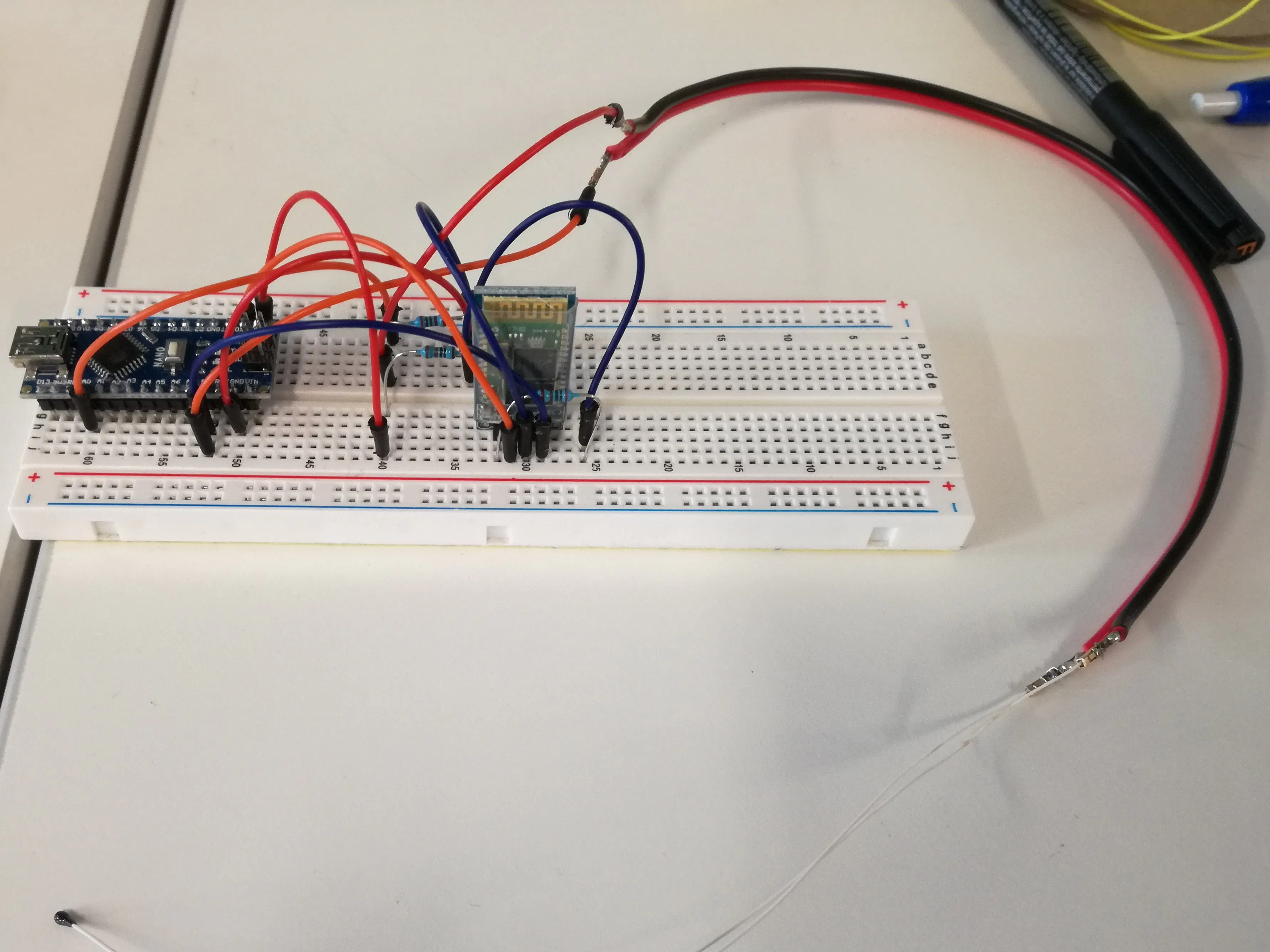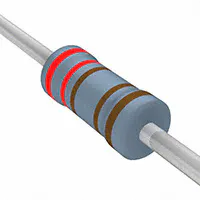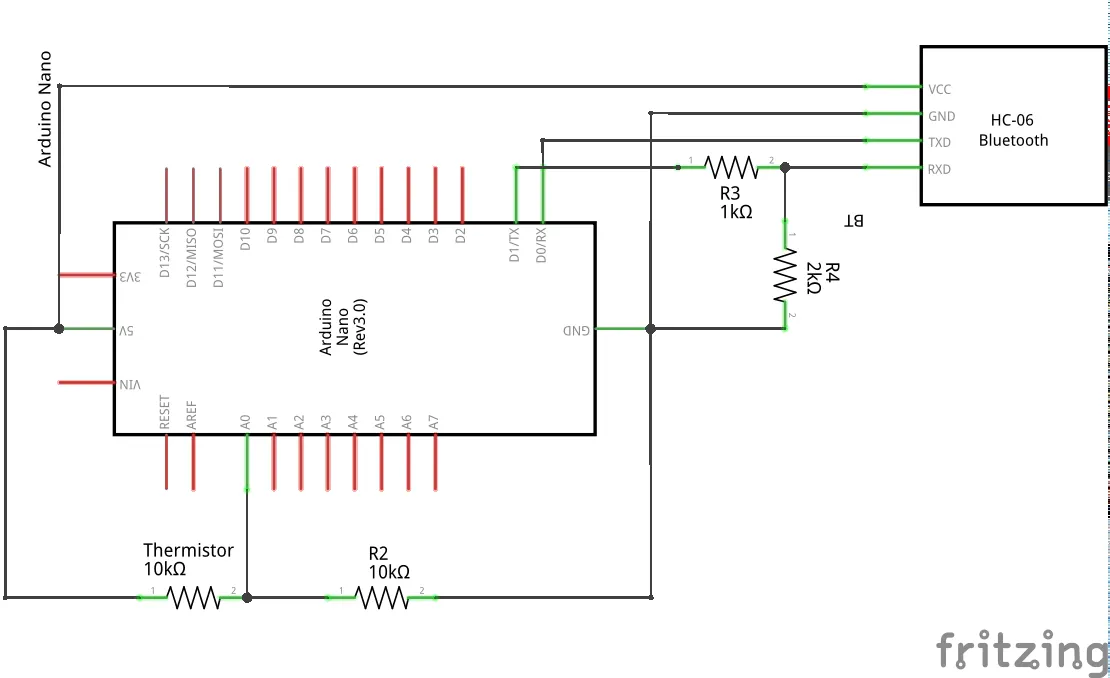# Bluetooth Temperature Monitor

Remote temperature monitor using a Bluetooth module and an NTC thermistor. An Android app made with App Inventor is used to read data.

BeginnerFull instructions provided2 hours4,893## Things used in this project

### Hardware componentsArduino Nano R3
×1Jumper wires (generic)
×1
 HC-06 Bluetooth Module
×1
 ntc thermistor
×1Resistor 10k ohm
×1Resistor 1k ohm
×1Resistor 2.21k ohm
×1

### Software apps and online servicesMIT App Inventor 2

### Hand tools and fabrication machinesSoldering iron (generic) Optional3D Printer (generic) Optional

## Schematics

### Arduino Schematics

Resistance R2 should be changed accordingly to the resistance of the thermistor you are going to use. The error in temperature reading increase with the bias (Runknow - R2).## Code

### Temperature monitor with bluetooth module

Arduino
In order to calculate the resistance of the NTC thermistor, 5V are applied to it in series with a know resistor of roughly the same magnitude ( in this example 10kohm). Through the analog pin A0 the voltage of the voltage divider made from the series resistance is calculated and using the known value of one resistance, the NTC resistance is calculated. This value is then converted to temperature using the beta equation (change the beta to the one you are using) and sent to the Android app using the Bluetooth module.
The code can be changed to monitor specific range of temperature, in this example I needed to monitor between 25 and 75°C and wait 5 minutes at the higher temperature.
```// Temperature monitor with bluetooth module

int analogPin= 0;
int raw= 0;
int Vin= 5;
float Vout= 0;
float R1= 10000; //change to your system
float Ro= 10000; //change to your system
float R2= 0;
float ratio= 0;
float Temp = 0;
float Beta = 3694; //change to your system
float To = 298.15;

// thermistor goes from 10k at 25°C. Choose reference resistance accondingly

void setup()
{
Serial.begin(9600);
}

void loop()
{
if(raw) {
ratio = raw * Vin;
Vout = (ratio)/1024.0;
ratio = (Vin/Vout) -1;
R2= R1 * ratio;
Temp = Beta/log(R2/(Ro*exp(-Beta/To)));
Temp = Temp -273.15;
if(Temp >= 74) {
for (int i = 0; i <= 30; i++) {
Serial.print("Temperature >= 74 °C"); //change to your system
delay(10000);
}
Serial.print("Heating Complete");
delay(10000);
} else if (Temp <= 26){
Serial.print("Temperature <= 25 °C"); //change to your system
delay(10000);
} else {
Serial.print("Temperature: "); //change to your system
Serial.println(Temp);
delay(10000);
}
}
}
```

## Credits

### Federico Vivaldi

5 projects • 22 followers
I'm a PhD student in chemistry and material science. I use my hobby in simple electronic to build items for laboratory.